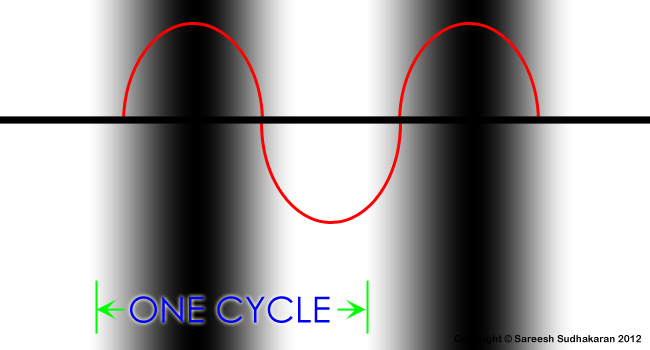# Spatial Frequency

Topics Covered:

• Spatial Frequency
• Cycles vs Lines vs Dots per mm

Why does the eye see a thing more clearly in dreams than the imagination when awake? – Leonardo da Vinci

Very simply put, the number of lines that fit a certain space defines the spatial frequency of the lines. If one can cram in a hundred lines in one centimeter, the spatial frequency is 100 lines per centimeter.

In optical measurements, the ‘lines’ are sometimes sine-wave gratings, so we don’t use the word ‘lines’. The sine-wave gratings (which can look like lines at high frequencies) are called cycles – there’s a rise and a fall.It is incorrect mathematically to use the term cycle when one is measuring lines, and vice versa. Yet, people do it everyday and cause a lot of confusion.

In optical imaging, since the gratings are often separated by lengths in the order of millimeters, the unit used for measurement is cycles per millimeter. If one is measuring lines instead, the unit used is lines per millimeter or line pairs per millimeter (a line pair is one white and one black). These are three different things entirely. Anyone using these terms interchangeably is doing everyone else a great disservice. Stay away from such people.

The number of sine-wave gratings that can be crammed into a millimeter of space is the spatial frequency of the gratings in cycles per millimeter. In precise measurements involving eyes, scientists sometimes use cycles per degree (or cycles per radian) to measure the angular spatial frequency. This is used because, traditionally, optical charts were based on angular resolution units like arc minutes or arc seconds.

The number of lines that can be crammed into a millimeter of space (it can all be one color) is the spatial frequency of the lines in lines per millimeter. In our daily activities and measurements, we substitute lines per mm for optotypes in eye exams, pixel counts on displays, dots per inch in printing, etc. I have to point out that a dot has its own mathematical function, called the point-spread function.

The number of line pairs that can be crammed into a millimeter of space (black and white with sharp edges) is the spatial frequency of the lines in line pairs per millimeter. Lens manufacturers, and sometimes television engineers, use this terminology or its variant.

All three are valid when used within their respective definitions. Measurement units are selected based on convenience and efficiency, and there is no absolute perfect standard that encompasses all visual sciences. Don’t forget that.

Takeaways:

• The Spatial Frequency of a system is the number of sine components that repeat over a given distance.
• Cycles, lines and dots are three different things, and depending on what one wishes to test or measure, the selection of units changes. With it, the mathematics that govern the exercise change as well. And you’ll get different results.